Function Repository Resource:

# IfSatisfies

Pass a value to a function if the value satisfies a condition

Contributed by: Roy Sianez
 ResourceFunction["IfSatisfies"][expr,condition,f,o] applies f to expr if condition[expr] evaluates to True; otherwise, applies o to expr.

## Details

expr can be any expression.
condition, f and o must all be functions or names of functions.

## Examples

### Basic Examples (1)

Produce a String telling whether a number is even or odd:

 In:=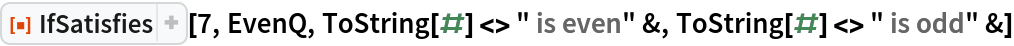Out=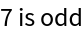### Scope (3)

The condition, as well as f and o, can be the name of a function:

 In:=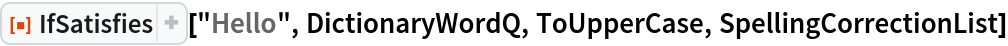Out=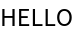In:=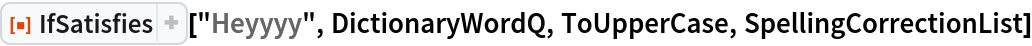Out=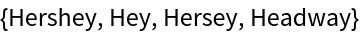Or they can be pure functions, or a mixture of both names of functions and pure functions:

 In:=Out=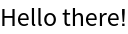Symbolic expressions are allowed. This example applies f to the expression if it has two items; otherwise, it applies g:

 In:=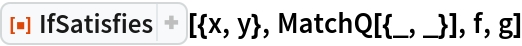Out=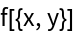### Applications (1)

If a word has conjugations or forms other than itself, return them; otherwise, return Missing[]:

 In:=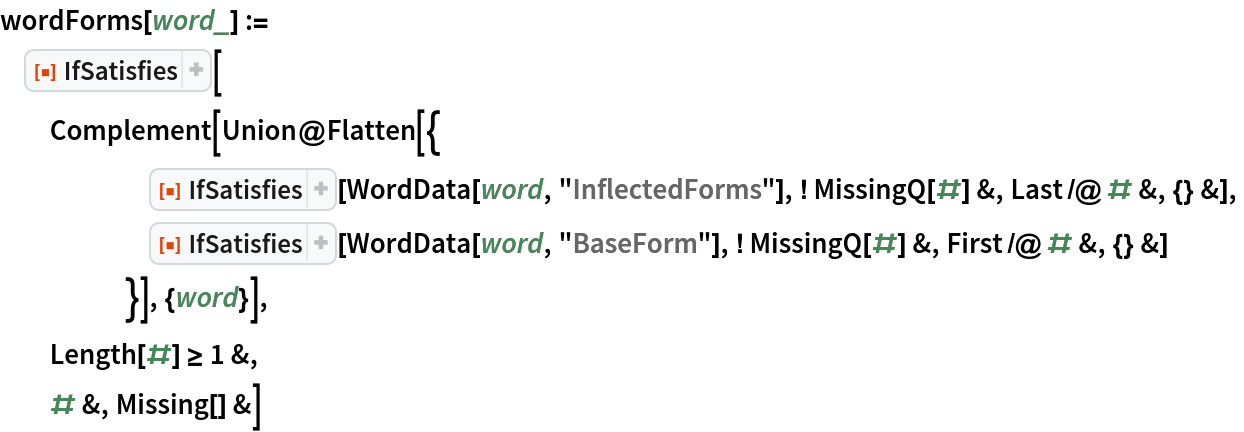In:=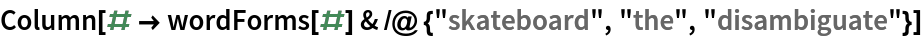Out=### Properties and Relations (1)

If the condition evaluates to something other than True or False, the "otherwise" function (the last argument) is evaluated. Here, the condition returns an image of a hot dog:

 In:=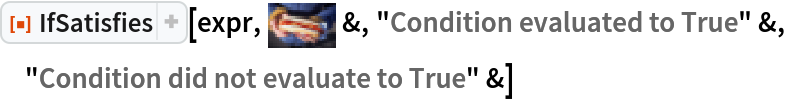Out=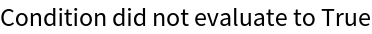### Possible Issues (2)

All arguments to this function except the first are required to be functions. Therefore, the following will not work:

 In:=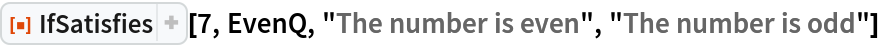Out=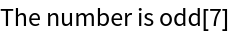Rather, make the arguments into pure functions like so:

 In:=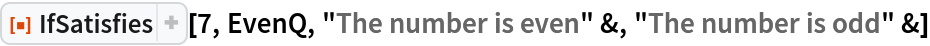Out=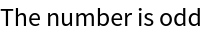Roy Sianez

## Version History

• 1.0.0 – 03 February 2021

## Author Notes

Inspired by the "if let" construct in the Swift programming language.